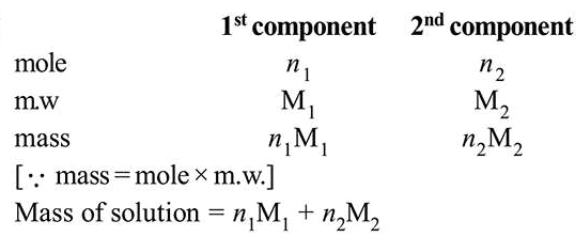# A solution of two components containing

Question:

A solution of two components containing $n_{1}$ moles of the $1^{\text {st }}$ component and $n_{2}$ moles of the $2^{\text {nd }}$ component is prepared. $M_{1}$ and $M_{2}$ are the molecular weights of component 1 and 2 respectively. If $d$ is the density of the solution in $g \mathrm{~mL}^{-1}, \mathrm{C}_{2}$ is the molarity and $x_{2}$ is the mole fraction of the $2^{\text {nd }}$ component, then $C_{2}$ can be expressed as:

1. $\mathrm{C}_{2}=\frac{1000 x_{2}}{\mathrm{M}_{1}+x_{2}\left(\mathrm{M}_{2}-\mathrm{M}_{1}\right)}$

2. $\mathrm{C}_{2}=\frac{d x_{2}}{\mathrm{M}_{2}+x_{2}\left(\mathrm{M}_{2}-\mathrm{M}_{1}\right)}$

3. $\mathrm{C}_{2}=\frac{1000 d x_{2}}{\mathrm{M}_{1}+x_{2}\left(\mathrm{M}_{2}-\mathrm{M}_{1}\right)}$

4. $\mathrm{C}_{2}=\frac{d x_{1}}{\mathrm{M}_{2}+x_{2}\left(\mathrm{M}_{2}-\mathrm{M}_{1}\right)}$

Correct Option: , 3

Solution:Mole fraction of the $2^{\text {nd }}$ component $\left(x_{2}\right)=\frac{n_{2}}{n_{1}+n_{2}}$

$\Rightarrow n_{1}=\frac{n_{2}\left(1-x_{2}\right)}{x_{2}}$

Mass of solution $=n_{1} \mathrm{M}_{1}+n_{2} \mathrm{M}_{2}$

$=\frac{n_{2} \mathrm{M}_{1}\left(1-x_{2}\right)}{x_{2}}+n_{2} \mathrm{M}_{2}$

$=\frac{n_{2}}{x_{2}}\left[\mathrm{M}_{2} x_{2}-x_{2} \mathrm{M}_{1}+\mathrm{M}_{1}\right]$

Volume of solution $=\frac{n_{2}\left[\mathrm{M}_{2} x_{2}-x_{2} \mathrm{M}_{1}+\mathrm{M}_{1}\right]}{1000 \mathrm{~d} x_{2}}$

$\mathrm{C}_{2}=\frac{1000 n_{2} \mathrm{~d} x_{2}}{n_{2}\left[\mathrm{M}_{2} x_{2}-x_{2} \mathrm{M}_{1}+\mathrm{M}_{1}\right]}$

$\Rightarrow \mathrm{C}_{2}=\frac{1000 \mathrm{~d} x_{2}}{\mathrm{M}_{1}+x_{2}\left(\mathrm{M}_{2}-\mathrm{M}_{1}\right)}$